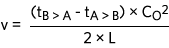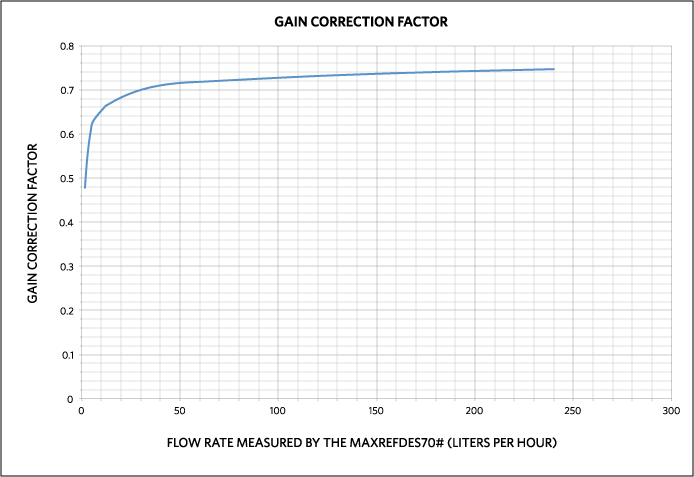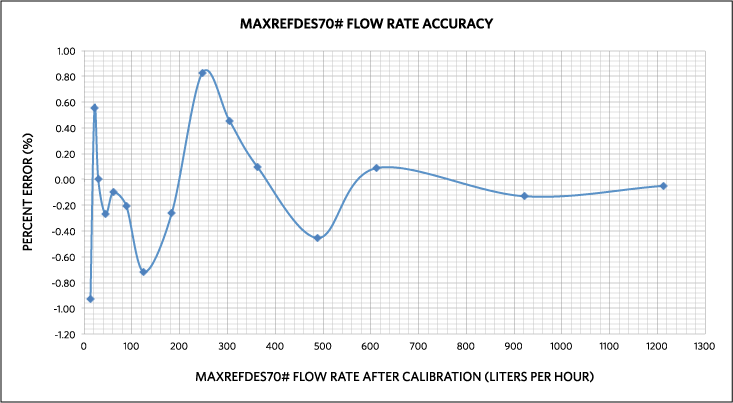# Calibration of Water Flow Rate in an Ultrasonic Flow Meter Using the MAX35101

### Abstract

The MAX35101 is a time-to-digital converter with built-in amplifier and comparator targeted as a complete analog front-end (AFE) solution for the ultrasonic heat meter and flow meter markets. With a time measurement accuracy of 20ps and automatic differential time-of-flight (TOF) measurement, this device makes for simplified computation of liquid or gas flow. Because of the effects of flow turbulence and mechanical design limitations in the spool body, the actual flow-rate measurement accuracy is limited and can only approach precise accuracy with averaging, flow compensation and multipoint calibration. This application note focuses on the actual procedure for performing the calibration of the flow meter.

#### Introduction

The flow rate measurement that occurs in an ultrasonic water meter is critical to the accuracy of the meter. All water meters built upon the transit-time ultrasonic principle require some kind of flow calibration. With the deployment of a reference standard flow measurement device, the meter can be calibrated across a specific known flow range. The calibration technique used for the set of measurements taken in this application note to obtain the multipoint compensation curve is based upon an electromagnetic reference flow meter that is traceable to the NIST gravimetric reference system. Calibration of each meter is unique, and usually performed by the meter manufacturer before shipment to the end customer. In the example within, a 22-point compensation curve is created for the meter and then the linear interpolation method is deployed to yield actual flow rates from the data measured by the meter. The accuracy of the newly calibrated meter is then measured by comparing the reference meter flow rate to the meter flow rate in a system where a single flow is measured by both the meter and the reference flow meter.

#### Why is Calibration Needed?

It has been shown that effects of nonidentical piezoelectric transducers on reciprocity and zero-flow calibration will always be present in a flow meter that uses the transit-time ultrasonic principle for flow measurement2, 3. The dynamic properties of fluids including viscosity influence the distribution of the velocity in the pipe create nonlinear behavior3. Process temperature and pressure lead to mechanical deformation of the spool body1. With proper calibration, the uncertainty of these various effects can be minimized so that the individual meter is made to operate within specifications dictated by various standards bodies worldwide.

#### Ultrasonic TOF Flow-Measurement Principle

A typical ultrasonic TOF water-flow meter spool body is depicted below in Figure 1.Figure 1. Typical ultrasonic TOF water-flow meter spool body.

The dimensions L and D are unique for every pipe size. The arrangement of the reflector surfaces within the flow spool body is also unique to every meter manufacturer. Consequently, the ultrasonic TOF principle is based upon the TOF of an acoustic signal in the water. The upstream TOF is longer than the downstream TOF, since the water velocity aids the acoustic signal in the downstream direction and impedes the acoustic signal in the upstream direction. This difference in TOF can be exploited to determine the velocity of the flowing water.

The acoustic TOF of an ultrasonic signal in the downstream direction is:

 t(A>B) = (D/2)/CO + L/(CO + v) + (D/2)/CO (Eq. 1)

The acoustic TOF of an ultrasonic signal in the upstream direction is:

 t(B > A) = (D/2)/CO + L/(CO + v) + (D/2)/CO (Eq. 2)

where:
CO is the speed of sound in water
v is the velocity of the water

Subtracting Equation 2 from Equation 1 and simplifying the results, followed then by solving for v, yields the velocity of the water:(Eq. 3)

The volumetric flow of the water is then simply calculated with knowledge of the cross-sectional area of the flow diameter of the spool body:(Eq. 4)

This volumetric flow-rate measurement is the basis for determining the flow rate in any flow system using transit-time ultrasonic principles.

#### Flow-Rate Calibration of the MAXREFDES70# High-Precision, Long-Battery-Life Heat/Flow Meter

Overview
The MAXREFDES70#, a Maxim Integrated reference design incorporating the MAX35101, is connected to a water-flow system so that the MAX35101 can actually measure flow rate through the meter spool body. The block diagram for MAX35101 is shown in Figure 2.Figure 2. Block diagram of the MAX35101 time-to-digital converter with analog front-end.

The design is composed of a MAX35101 mated to a low-power microcontroller, a battery, the spool body, and the transducers. The MAX35101 can take automatic differential TOF measurements. Its Early Edge Detect feature allows the MAX35101 to measure zero-crossing data consistently between measurements. With its ability to measure the temperature of the water, the TOF data can be compensated for the differences in the speed of sound in water at different temperatures. The actual value of CO can be calculated. The MAX35101 offers an event timing mode that is configurable and runs cyclic algorithms to minimize microprocessor interactivity and increases battery life. The real-time clock (RTC) provides one programmable alarm and watchdog functionality. A simple opcode-based 4-wire SPI interface allows any microcontroller to effectively configure the device for its intended measurement. On-board user flash allows the MAX35101 to be nonvolatile configurable and provides nonvolatile energy use data to be logged. A full system diagram of MAXREFDES70# using the MAX35101 is shown in Figure 3.Figure 3. MAXREFDES70# using the MAX35101 time-to-digital converter with analog front-end.

The data needed to calibrate the MAXREFDES70# is collected by also installing a highly accurate flow-rate reference. The National Institute of Standards and Technology (NIST) in the United States uses a gravimetric reference system. This is a weigh system with a collection tank and a flow-diverting device. This device is the part of the calibration system that directs the flowing water into the collection tank while triggering a clock to determine the collection time. The water collected can be determined in terms of volumetric or gravimetric units. More information on this method of calibration can be obtained at the NIST website.

The calibration technique used for the set of measurements taken to obtain the multipoint compensation curve is based upon an electromagnetic reference flow meter that is traceable to the NIST gravimetric reference system.To meet the OIML R49/EN14154 specifications for a Class II water meter, the flow rate must be ±5% accurate below the meter Q2 specification, and ±2% for flow rates up to the meter Q4 specification. The rule of thumb for calibration is that the reference should be four times more accurate than the unit under test. The chosen reference meter for the calibration of the MAXREFDES70# in this exercise has a flow rate accuracy of ±0.2% down to a flow rate of 14 liters/hour. It must be stated that when taking flow rate data from the MAXREFDES70# and the reference meter, the flow must be in a steady-state. Since the flow rate of the reference meter and the MAXREFDES70# are compared in real time during this calibration, the system flow rate should not vary with time. All of the water that is measured by the reference meter must also be measured by the MAXREFDES70#.

Calibration Procedure
Calibration of each meter is unique, and usually performed by the meter manufacturer before shipment to the end customer. In this exercise, the MAXREFDES70# is calibrated using a 22-point compensation curve, although with experimentation, a smaller set of calibration points may be enough to meet the needs of your application.

As mentioned, data at 22 different calibration flow rates is taken by comparing the reference flow meter reading (actual) to the MAXREFDES70# measured flow (measured). An example of such a data set is shown in Table 1. The calibration flow rates chosen are concentrated near the lower flow range of the MAXREFDES70#. This is the flow range most affected by the issues described in the Why is Calibration Needed? section.

Table 1. Collected Calibration Data
Calibration Points Actual Reference (Liters per Hour) measured by MAXREFDES70 (Liters per Hour) Gain Correction Factor
1 0.7171706 1.5 0.47811375
2 1.660545 3 0.553515
3 2.7184669 4.5 0.60410375
4 3.77928 6 0.62988
5 7.9496736 12 0.6624728
6 8.9999931 13.5 0.666666155
7 10.059539 15 0.670635938
8 11.127412 16.5 0.674388627
9 12.202753 18 0.6779307
10 14.372587 21 0.684408913
11 16.562938 24 0.6901224
12 18.768321 27 0.695122988
13 20.983875 30 0.6994625
14 23.205361 33 0.703192763
15 25.429162 36 0.7063656
16 29.872345 42 0.7112463
17 34.296922 48 0.7145192
18 38.696341 54 0.7165989
19 43.074 60 0.7179
20 87.737524 120 0.731146033
21 133.22772 180 0.740153989
22 179.18699 240 0.746612461

Also included in Table 1 is the gain correction factor for each of the reference flow rates measured. This gain correction factor is computed as actual ÷ measured and represents the gain that needs to be applied to the flow rate measured by MAXREFDES70# so that is matches the reference flow meter. A plot of this gain factor is shown in Figure 4.Figure 4. Gain correction factor vs. measured MAXREFDES70# flow rate.

The firmware in the MAXREFDES70# requires the information from Table 1 to compute the actual flow rate from the measured flow rate. In particular, the calibration table for the firmware is a 22 x 2 array of the measured MAXREFDES70# flow rate vs. the gain correction factor.

Actual Flow Computation
The actual flow rate is computed using the method of linear interpolation. The flow rate is measured by the MAXREFDES70#. This is the value vmfrom Equation 4. The calibration table is searched to find the two entries that bound X. Let these two values be X0 and X1 such that X1 > X > X0. The corresponding values for Y1 and Y0 are then extracted from the adjacent column that represents the gain correction factor. Then, the gain correction factor corresponding to the flow rate measured by the MAXREFDES70# is computed as Y:

Y = Y0 + (Y1 - Y0) × (vm - X0)/(X1 - X0)

The actual flow rate is then computed as vf :

vf = vm × Y

Rate Accuracy
The accuracy of the newly calibrated MAXREFDES70# can then be measured by comparing the reference meter flow rate to the MAXREFDES70# flow rate in a system where a single flow is measured by both the MAXREFDES70# and the reference flow meter. The results of the accuracy are shown in Figure 5.Figure 5. MAXREFDES70# calibrated accuracy.

It can be seen that the MAXREFDES70# accuracy is never worse than ±1% all the way down to the minimum flow measured in Figure 5 of 12.9 LPH.

#### Conclusion

Calibration of an ultrasonic flow meter requires the use of an NIST-traceable reference flow meter. The accuracy of the MAXREFDES70# can only approach the accuracy of the reference flow meter. With correct selection of the reference flow meter and the correct calibration procedure, the MAXREFDES70# can easily be made to meet the OIML R49/EN14154 specifications for water meters. The accuracy of the MAXREFDES70# calibrated in this exercise is better than ±1% down to a low flow rate of 12.9 LPH.

References

1. Karsten Tawackolian, Oliver Büker, Jankees Hogendoorn, Thomas Lederer. "Calibration of an ultrasonic flow meter for hot water." Physikalisch-Technische Bundesanstalt (PTB), Heat and Vacuum department, Abbestraße 2-12, 10587 Berlin, Germany KROHNE Altometer, Kerkeplaat 12, 3313LC Dordrecht, The Netherlands.
2. J. Hogendoorn, H. Hofstede, P. van Brakel, A. Boer. "How accurate are ultrasonic flowmeters in practical conditions; beyond the calibration." 29th International North Sea Flow Measurement Workshop: pp. 25-28. Tonsberg, Norway, October 2011.
3. J. van Deventer, J. Delsing. "Apparent transducer non-reciprocity in an ultrasonic flow meter." Ultrasonics, Vol. 40 (Issues 1-8) (2002), pp. 403-405.